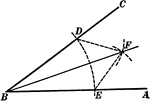### Construction Of Angle Bisector

Illustration showing how to construct the bisector of an angle.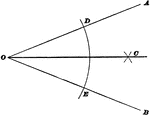### Bisect An Angle

Illustration used to show how to construct a bisector of an angle when the sides intersect within the…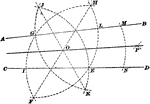### Bisect An Angle

Illustration used to show how to construct a bisector of an angle when the sides no not intersect within…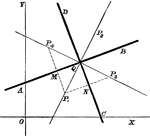### Line Bisectors

Multiple lines showing many bisectors on the coordinate plane.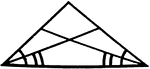### Angle Bisectors In An Isosceles Triangle

Illustration showing that if two angles of a triangle are equal, the bisectors of these angles are equal.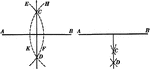### Construction Of A Perpendicular Bisector Of A Straight Line

Illustration of the construction used to make a perpendicular bisector of a straight line.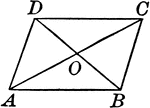Illustration to show that if the diagonals of a quadrilateral bisect each other, the figure is a parallelogram.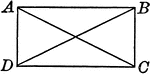### Rectangle With Diagonals

Illustration to show that if the diagonals of a parallelogram are equal, the figure is a rectangle.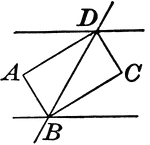### Rectangle Formed When Two Parallel Lines are Cut by a Transversal

Illustrations to show that if two parallel lines are cut by a transversal, the bisectors of the interior…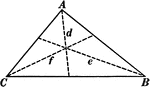### Angle Bisectors In A Triangle

Illustration showing the three angle bisectors in a triangle.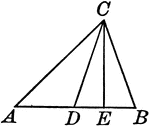### Triangle With Bisector and Perpendicular From Vertex Drawn

Illustration to show the bisector of the vertical angle of a triangle makes with the perpendicular from…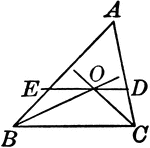### Triangle With Bisectors of Base Angles

Illustration to show if the bisector of the base angles of a triangle are drawn, and through their point…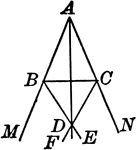### Triangle With Bisectors of Vertical Angles

Illustration to show the bisector of the vertical angle A of a triangle ABC, and the bisectors of the…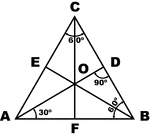### Equilateral Triangle

Sketch of equilateral triangle with bisectors, medians, and altitudes.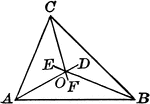### Incenter of Triangle

Illustration to show angle bisectors in a triangle. It is known as the incenter.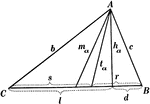### Parts Of A Triangle

Illustration used to show the various parts of a triangle: sides, angles, medians, altitudes, bisectors,…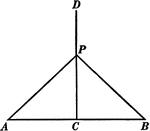### Triangle With Perpendicular Bisector

Illustration showing a perpendicular bisector of a triangle extended outside of the triangle.